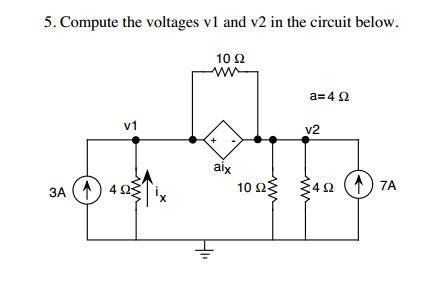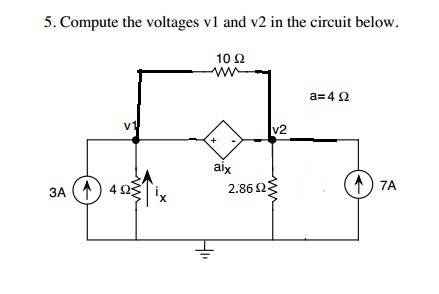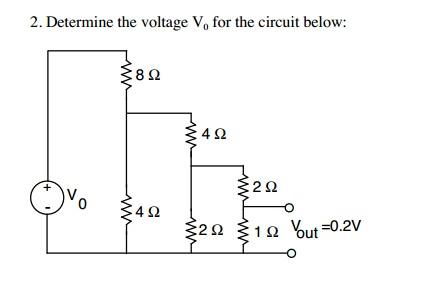# Node Voltage Analysis Questions

turpy
Hopefully it's ok if I post two questions

QUESTION 1

## Homework Statement## Homework Equations

Node voltage analysis method

## The Attempt at a Solution

First of all is this circuit equivalent?(combining the 10 ohm and 4 ohm in parallel, and extending the ends of the protruding 10 om to nodes for v1 and v2?)

If so I solve (using the sum of all currents flowing into a node =0)
(1) 3 + ix + (v2-v1)/10 + 4*ix =0
(2) -4*ix + 7 + (v1-v2)/10 - v2 /2.86 = 0
with ix = -v1 /4
(1) -1.35*v1 + 0.1*v2 = -3
(2) 1.1*v1 - 0.45*v2 = -7
v1 = 4.12 V
v2 = 25.63 V

QUESTION 2

## Homework Statement## Homework Equations

node voltage analysis method

## The Attempt at a Solution

I'm at a loss as to where I should place the reference ( 0V ground) voltage, and where to put the nodes for node voltage analysis.

Typically the ground is at the negative terminal of the voltage source. But if I put it there, would the other junctions along the bottom be 0V? I thought the voltage drops would be the same only if the resistors were in parallel, and with the ladder/steps I'm not so sure.

We're given that there's 0.2V across the 1ohm resistor, so it's 2A of current flowing through it by ohm's law. The 2 ohm resistor above is has the same 2A flowing through since it's in series. But does the 2 ohm resistor in parallel (are they parallel?) to them have 2A flowing through, and then in turn the 4 ohm resistor?
I'm really missing something to understand how currents flow and voltages drop in a circuit

Thanks!